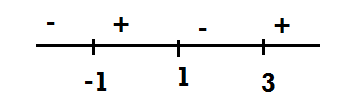Filters

Q&A - Ask Doubts and Get Answers
Q

Try this! - Algebra - BITSAT-7

The solution set of  is

• Option 1)

• Option 2)

• Option 3)

• Option 4)

None.

105 Views

As learnt in concept

Range -

The range of the relation R is the set of all second elements of the ordered pairs in a relation R.

- wherein

eg. R={(a,b),(c,d)}. Then Range is {b,d}

and wavy curves,Option 1)

This option is incorrect.

Option 2)

This option is correct.

Option 3)

This option is incorrect.

Option 4)

None.

This option is incorrect.

Exams
Articles
Questions